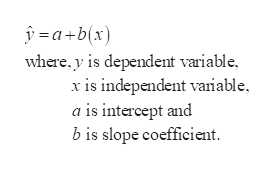# How can I calculate the different values in the equation y=ax+b for the regression line of a sample? How can I find the value for a and the value for b?

Question
1 views

How can I calculate the different values in the equation y=ax+b for the regression line of a sample? How can I find the value for a and the value for b?

check_circle

Step 1

The form of the simple linear ...help_outlineImage Transcriptionclosea+b(x) where, y is dependent variable x is independent vaniable a is intercept and bis slope coefficient fullscreen

### Want to see the full answer?

See Solution

#### Want to see this answer and more?

Solutions are written by subject experts who are available 24/7. Questions are typically answered within 1 hour.*

See Solution
*Response times may vary by subject and question.
Tagged in
MathStatistics

### Hypothesis Testing